Courses

# Olympiad Test: Mirror Images - 1

## 10 Questions MCQ Test Mathematics Olympiad Class 7 | Olympiad Test: Mirror Images - 1

Description
This mock test of Olympiad Test: Mirror Images - 1 for Class 7 helps you for every Class 7 entrance exam. This contains 10 Multiple Choice Questions for Class 7 Olympiad Test: Mirror Images - 1 (mcq) to study with solutions a complete question bank. The solved questions answers in this Olympiad Test: Mirror Images - 1 quiz give you a good mix of easy questions and tough questions. Class 7 students definitely take this Olympiad Test: Mirror Images - 1 exercise for a better result in the exam. You can find other Olympiad Test: Mirror Images - 1 extra questions, long questions & short questions for Class 7 on EduRev as well by searching above.
QUESTION: 1

Solution:
QUESTION: 2

Solution:
QUESTION: 3

### Direction: In each of the following questions you are given a combination of alphabets and/or numbers followed by four alternatives (1), (2), (3) and (4). Q. Choose the alternative which closely resembles the mirror-image of the given combination.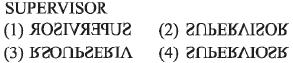Solution:
QUESTION: 4

Direction: In each of the following questions you are given a combination of alphabets and/or numbers followed by four alternatives (1), (2), (3) and (4).
Q. Choose the alternative which closely resembles the mirror-image of the given combination.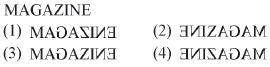Solution:
QUESTION: 5

Direction: In each of the following questions you are given a combination of alphabets and/or numbers followed by four alternatives (1), (2), (3) and (4).
Q. Choose the alternative which closely resembles the mirror-image of the given combination.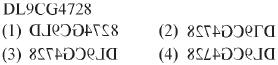Solution:
QUESTION: 6

Direction: In each of the following questions you are given a combination of alphabets and/or numbers followed by four alternatives (1), (2), (3) and (4).
Q. Choose the alternative which closely resembles the mirror-image of the given combination.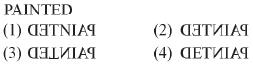Solution:
QUESTION: 7

Direction: In each of the following questions you are given a combination of alphabets and/or numbers followed by four alternatives (1), (2), (3) and (4).
Q. Choose the alternative which closely resembles the mirror-image of the given combination.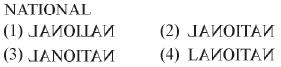Solution:
QUESTION: 8

Direction: In each of the following questions you are given a combination of alphabets and/or numbers followed by four alternatives (1), (2), (3) and (4).
Q. Choose the alternative which closely resembles the mirror-image of the given combination.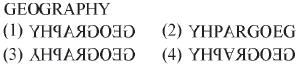Solution:
QUESTION: 9

Direction: In each of the following questions you are given a combination of alphabets and/or numbers followed by four alternatives (1), (2), (3) and (4).
Q. Choose the alternative which closely resembles the mirror-image of the given combination.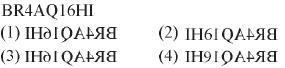Solution:
QUESTION: 10

Direction: In each of the following questions you are given a combination of alphabets and/or numbers followed by four alternatives (1), (2), (3) and (4).
Q. Choose the alternative which closely resembles the mirror-image of the given combination.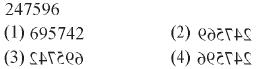Solution: